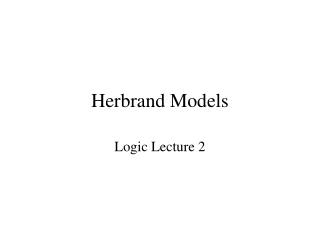# Herbrand Models - PowerPoint PPT PresentationDownload PresentationHerbrand Models

Herbrand Models
Download Presentation## Herbrand Models

- - - - - - - - - - - - - - - - - - - - - - - - - - - E N D - - - - - - - - - - - - - - - - - - - - - - - - - - -
##### Presentation Transcript

1. Herbrand Models Logic Lecture 2

2. Example: Models X(Y((mother(X)  child_of(Y,X))  loves(X,Y))) mother(mary) child_of(tom,mary)

3. Problem… • Difficult to compare two interpretations with different domains… e.g., one domain consists of apples and the other of oranges. • Could map one domain to another. Can be tricky to define… most domains are infinite. • Idea: for a given alphabet, pick a canonical domain and mapping. But how?

4. Some Notes • Typically, we are given a theory (set of sentences) T and wish to speak of Herbrand interpretations relative to T. • In this case we take the alphabet A to be the symbols in T. • If T has no constants, we introduce one.

5. Notes (continued) • Valuations with respect to a Herbrand interpretation may be thought of as grounding substitutions. • We’d like know it’s sufficient to consider only Herbrand interpretations… just ignore all others…

6. Herbrand Model Lemma • Let T be a theory (set of sentences) in Skolem Normal Form. • T has a model iff it has a Herbrand model.

7. Skolemization • Process is applied to one sentence at a time and applied only to the entire sentence (so outermost quantifier first). Each sentence initially has empty vector of free variables. • Replace X A(X) with A(X), and add X to vector of free variables. • Replace X A(X) with A(x(V)) where x is a new function symbol and V is the current vector of free variables.

8. Herbrand Model Lemma • Let T be a theory (set of sentences) in Skolem Normal Form. • T has a model iff it has a Herbrand model. Now recall our goal of identifying a unique simplest model.

9. Example: Models X(Y((mother(X)  child_of(Y,X))  loves(X,Y))) mother(mary) child_of(tom,mary)

10. Why no least Herbrand model? • Disjunctive “positive” information… creates uncertainty. We can satisfy the disjunction by satisfying either disjunct – a choice. • This is somewhat analogous to the uncertainty created by existential quantifiers. • This uncertainty also causes inefficiencies in deduction (recall prop. SAT is NP-complete but SAT for Horn CNFs is linear-time solvable).

11. Examples of Definite Programs mother(mary) child_of(tom,mary) loves(X,Y) mother(x)  child_of(X,Y) odd(s(0)) odd(s(s(X)) odd(X)

12. Prolog Notation mother(mary). child_of(tom,mary). loves(X,Y):- mother(x), child_of(X,Y). odd(s(0)). odd(s(s(X)):- odd(X).

13. About Least Herbrand Models • The least Herbrand model MP of a program P is the set of all ground atomic logical consequences of the program. • In general it is undecidable whether a ground atomic formula is in the least Herbrand model of a program (logically follows from the program). But if it follows, it can be eventually shown…

14. Alternative Characterization of Least Herbrand Model • Let P be a definite program. TP is a function on Herbrand interpretations defined as follows: TP(I) = {A0 | A0A1,…,Am  Pgr and {A1,…,Am}  I} • The least interpretation I such that TP(I) = I is the least Herbrand model of P.

15. Contruction/Approximation of Least Herbrand Model • TP 0 =  • TP (i+1) = TP(TP i) • TPw is the union of TP i for all i from 0 to  • The least Herbrand model MP of P is the least fixpoint of TP: the least Herbrand interpretation such that TP(MP) = MP. • MP = TPw.

16. Example • odd(s(0)). • odd(s(s(X))  odd(X). • TP 0 =  • TP 1 = {odd(s)} • TP 2 = {odd(s(s(s(0))), odd(s)} • TPw = {odd(sn(0)) | n  {1,3,5,…}}